# Universal property

(diff) ← Older revision | Latest revision (diff) | Newer revision → (diff)

A property of an object in a category which characterizes it as a representing object for some (covariant or contravariant) set-valued functor defined on the category. More formally, let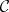be a category and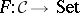a functor (for definiteness, the covariant case is treated here). Then a universal element ofis a pair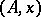, whereis an object ofand, such that for every other such pair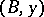there is a unique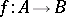insatisfying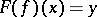. The correspondence betweenanddefines a natural isomorphism betweenand the functor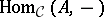; the objectis said to be a representing object (or representation) for the functor, and its universal property is the possession of the universal element.

### Examples.

1) In any category, the universal property of a (categorical) product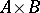is the possession of a pair of projections; that is,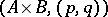is a universal element for the (contravariant) functor which sends an objectto the set of all pairs of morphisms.

2) In the category of modules over a commutative ring, the universal property of a tensor productis the possession of a bilinear mapping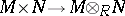; that is,is a representing object for the covariant functor which sends a moduleto the set of bilinear mappings.

An object possessing a given universal property is unique up to canonical isomorphism in the appropriate category. The idea of characterizing objects by means of universal properties was first exploited by S. MacLane [a1].

How to Cite This Entry:
Universal property. Encyclopedia of Mathematics. URL: http://encyclopediaofmath.org/index.php?title=Universal_property&oldid=17411
This article was adapted from an original article by P.T. Johnstone (originator), which appeared in Encyclopedia of Mathematics - ISBN 1402006098. See original article